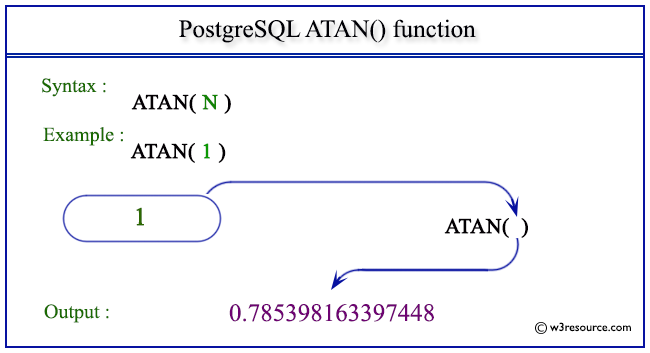# PostgreSQL ATAN() function

## ATAN() function

The PostgreSQL atan() function is used to return the inverse tangent of a given argument.

Syntax:

```atan(a)
```

PostgreSQL Version: 9.3

Pictorial presentation of PostgreSQL ATAN() functionExample 1: PostgreSQL ATAN() function

Code:

``````SELECT ATAN(0) AS "Atan (0)";
```
```

Sample Output:

``` Atan (0)
----------
0
(1 row)
```

Example 2: PostgreSQL ATAN() function

Code:

``````SELECT ATAN(1) AS "Atan (1)";
```
```

Sample Output:

```     Atan (1)
-------------------
0.785398163397448
(1 row)
```

Example 3: PostgreSQL ATAN() function

Code:

``````SELECT ATAN(2) AS "Atan (2)";
```
```

Sample Output:

```     Atan (2)
------------------
1.10714871779409
(1 row)
```

Previous: ASIN function
Next: ATAN2 function

﻿